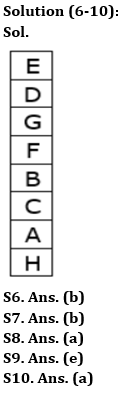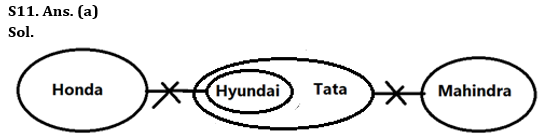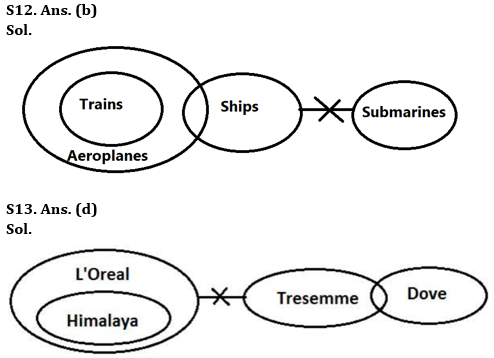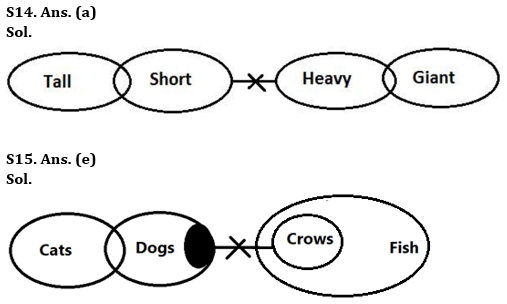Latest Banking jobs   »

# Reasoning Ability Quiz For Bank Foundation 2023 -06th May

Directions (1-5): In the given questions, relationship between different elements is shown in the statement(s). These statements are followed by two conclusions. Find which of the conclusion(s) will be definitely true.

Q1. Statements: L > M < N = R ≤ S, T ≥ G < W> S
Conclusions: I. R > T
II. M < W
(a) Only conclusion I is true
(b) Only conclusion II is true
(c) Either conclusion I or II is true
(d) Neither conclusion I nor II is true
(e) Both conclusions I and II are true

Q2. Statements: P ≥ Q, Q ≤ X, X > S, S < Y
Conclusions: I. P > X
II. Y > X
(a) Only conclusion I is true
(b) Only conclusion II is true
(c) Either conclusion I or II is true
(d) Neither conclusion I nor II is true
(e) Both conclusions I and II are true

Q3. Statements: N = K < E ≤ B = Q ≥ U > G
Conclusions: I. B > G
II. Q ≥ E
(a) Only conclusion I is true
(b) Only conclusion II is true
(c) Either conclusion I or II is true
(d) Neither conclusion I nor II is true
(e) Both conclusions I and II are true

Q4. Statements: L ≤ Q, N > Z, L ≤ Z, N = Y
Conclusions: I. L < Y
II. Z > Q
(a) Only conclusion I is true
(b) Only conclusion II is true
(c) Either conclusion I or II is true
(d) Neither conclusion I nor II is true
(e) Both conclusions I and II are true

Q5. Statements: W ≥ U = J, K ≤ V = S, S < M > W
Conclusions: I. M > J
II. V < W
(a) Only conclusion I is true
(b) Only conclusion II is true
(c) Either conclusion I or II is true
(d) Neither conclusion I nor II is true
(e) Both conclusions I and II are true

Directions (6-10): Study the following information carefully and answer the given questions.
Eight boxes i.e. A, B, C, D, E, F, G, and H are placed one above the other in a stack but not necessarily in the same order. Two boxes are placed between Box H and Box B. Box H is either placed at topmost or at bottommost position. Three boxes are placed between Box C and Box D which is placed above box B. One box is placed between Box D and Box F. One box is placed between Box E and Box G which is placed above Box A. More than three boxes are placed between Box A and Box E.

Q6. Which box is placed at 6th position from bottom?
(a) D
(b) G
(c) F
(d) B
(e) None of these

Q7. How many boxes are placed below Box A?
(a) Three
(b) One
(c) None
(d) Two
(e) More than three

Q8. How many boxes are placed between Box G and Box A?
(a) Three
(b) More than three
(c) None
(d) Two
(e) One

Q9. Four of the following five pairs are alike in a certain way and hence form a group. Find the one which does not belong to that group.
(a) H-A
(b) F-G
(c) D-E
(d) C-A
(e) G-H

Q10. If we arrange all the boxes according to English alphabetical series from bottom to top, then how many boxes will remain at same place?
(a) One
(b) Three
(c) Two
(d) Five
(e) None

Directions (11-15): In the question below three statements are given followed by two conclusions. You have to take the given statements to be true even if they seem to be at variance with commonly known facts. Read all the conclusions and then decide which of the given conclusions logically follows from the given statements disregarding commonly known facts.

Q11. Statements: No Honda is Hyundai.
All Hyundai are Tata.
No Tata is Mahindra.
Conclusion: I. A few Honda being Tata is possibility
II. No Mahindra is Honda
(a) Only conclusion I follows
(b) Only conclusion II follows
(c) Either conclusion I or II follows
(d) Both conclusions I and II follow
(e) Neither conclusion I nor II follows

Q12. Statements: Only Aeroplanes are trains.
No submarine is ship.
A few Ships are Aeroplanes.
Conclusions: I. A few Ships being Trains is a possibility
II. A few Aeroplanes are not submarines
(a) Only conclusion I follows
(b) Only conclusion II follows
(c) Either conclusion I or II follows
(d) Both conclusions I and II follow
(e) Neither conclusion I nor II follows

Q13. Statements: All Himalaya are L’Oreal.
No L’Oreal is Tresemme.
Only a few Tresemme are Dove.
Conclusions: I. No Himalaya is Tresemme
II. Some Dove are not L’Oreal
(a) Only conclusion I follows
(b) Only conclusion II follows
(c) Either conclusion I or II follows
(d) Both conclusions I and II follow
(e) Neither conclusion I nor II follows

Q14. Statements: Only a few Tall are Short.
No Short is Heavy.
Only a few Heavy are Giant.
Conclusions: I. Some Tall is not Heavy
II. No Giant is Short
(a) Only conclusion I follows
(b) Only conclusion II follows
(c) Either conclusion I or II follows
(d) Both conclusions I and II follow
(e) Neither conclusion I nor II follows

Q15. Statements: Only a few Cats are Dogs.
Some Dogs are not Crows.
All Crows are Fish.
Conclusions: I. Some Dogs are not Fish
II. Some Cats are not Fish
(a) Only conclusion I follows
(b) Only conclusion II follows
(c) Either conclusion I or II follows
(d) Both conclusions I and II follow
(e) Neither conclusion I nor II follows

Solutions

Solution (1-5):
S1. Ans. (b)
Sol. I. R > T – False
II. M < W – True

S2. Ans. (d)
Sol. I. P > X – False
II. Y > X – False

S3. Ans. (e)
Sol. I. B > G – True
II. Q ≥ E – True

S4. Ans. (a)
Sol. I. L < Y – True
II. Z > Q – False

S5. Ans. (a)
Sol. I. M > J – True
II. V < W – False## FAQs

### What is the selection process of the Bank Clerk?

The selection process of the Bank Clerk is Prelims & Mains.

#### Congratulations!Union Budget 2023-24: Free PDF